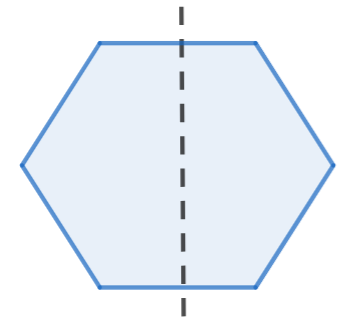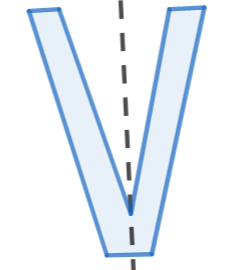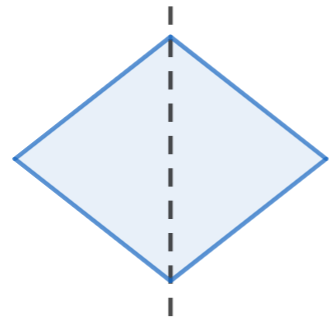Courses
Courses for Kids
Free study material
Offline Centres
MoreLast updated date: 04th Dec 2023
Total views: 281.4k
Views today: 6.81k

# Draw the lines of symmetry for the following figures.a.b.c.Verified
281.4k+ views
Hint: In this problem, we have to draw the symmetric line for the given diagram. We should know that a line of symmetry is a line that cuts a shape exactly in half. This means that if we fold the shape along the line, both halves would match exactly. We can now draw the symmetric line for each of the following given diagrams to solve this problem.

Here we have to draw the line of symmetry for the given diagrams.
We should know that a line of symmetry is a line that cuts a shape exactly in half. This means that if we fold the shape along the line, both halves would match exactly.
We can now draw the symmetric line for each of the following given diagrams.
We can first draw the symmetric line for the first figure, we get
1.We can see that, the line of symmetry, split the figure into equal halves, similarly for the figure 2 and 3,Note: We should always remember that a line of symmetry is a line that cuts a shape exactly in half. This means that if we fold the shape along the line, both halves would match exactly. We can cut the diagram into two equal halves using the symmetric line.